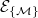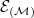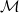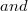﻿

### Characterization of ultradifferentiable test functions defined by weight matrices in terms of their Fourier Transform

#### Abstract

We prove that functions with compact support in non-quasianalytic classes$\mathcal{E}_{\{\mathcal{M}\}}$ of Roumieu-type and$\mathcal{E}_{(\mathcal{M})}$ of Beurling-type defined by a weight matrix$\mathcal{M}$ with some mild regularity conditions can be characterized by the decay properties of their Fourier transform. For this we introduce the abstract technique of constructing from$\mathcal{M}$ multi-index matrices and associated function spaces. We study the behaviour of this construction in detail and characterize its stability. Moreover non-quasianalyticity of the classes mathcal{E}_{\{\mathcal{M}\}}$and$\mathcal{E}_{(\mathcal{M})}\$ is characterized.

DOI Code: 10.1285/i15900932v36n2p1

Keywords: Ultradifferentiable functions; non-quasianalyticity; Fourier transform

Full Text: PDF